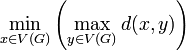## Definition

The radius of a graph is defined for any connected graph as the radius of the metric space induced by it. Explicitly, for a graphwith vertex set, it is:wheredenotes the distance between two vertices. In words, the radius of a graph is the minimum, over all vertices, of the eccentricity of that vertex.

Note that for a finite graph, the radius is finite. For an infinite graph, the radius may be finite or.

For a graph that is not connected, we can consider the radius to be eitheror undefined.

## Related invarants

Diameter of a graph maximum of distances between pairs of vertices RadiusDiameterTwice the radius